#mysql锁技术讨论

0
0
01. 云栖社区>
2. 博客>
3. 正文

# 2 要明确的概念

• 不可重复读和幻读的区别
• 快照读和当前读
• 事务的隔离级别
• record lock、gap lock、next-key lock

## 2.1 不可重复读和幻读的区别

select数据的不变性可以细分成2部分

• 第一部分就是：对原有数据的不可修改性，如update delete，通过行锁锁住记录就可以实现不可修改
• 另一部分就是：对于新增数据的限制，这时候就不能通过行锁来解决了，这时候就需要通过gap lock来解决

## 2.3 事务的隔离级别

• 对于快照读中的幻读（即select * from t where id>=6出现的幻读）采用的解决方式是采用MVCC（多版本并发控制）
• 对于当前读中的幻读（即select * from t where id>=6 for update出现的幻读）采用的解决方式是gap lock

# 3 问题分析

``````start transaction;
DELETE FROM t WHERE id =6;
INSERT INTO t VALUES(6);
commit;
``````

• 使用了什么锁？阻塞情况？
• 是否会出现死锁？

``````create table m (
id int ,
primary key (id)
);
``````

``````insert into m values(1),(2),(6),(8);
``````

``````start transaction;
delete from m where id =6;
``````

``````start transaction;
delete from m where id =6;
``````

``````insert into m value(6);
``````

``````insert into m value(6);
``````

### 3.3.1 删除一个已存在的值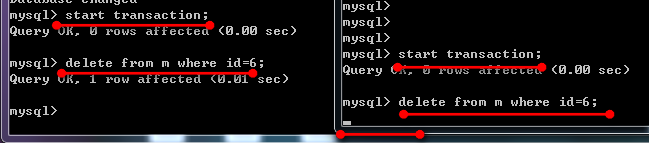### 3.3.2 删除一个不存在的值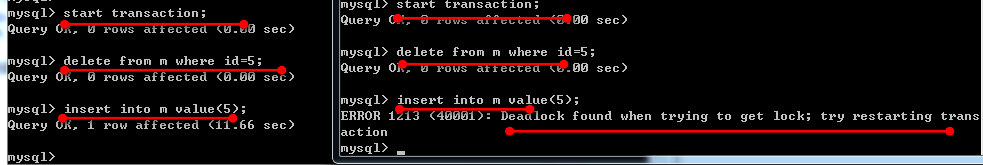``````create table t (
id int ,
key (id)
);
``````

``````insert into t values(1),(2),(6),(8);
``````

``````start transaction;
delete from t where id =6;
``````

``````start transaction;
delete from t where id =6;
``````

``````insert into t value(6);
``````

``````insert into t value(6);
``````

### 3.4.1 删除一个已存在的值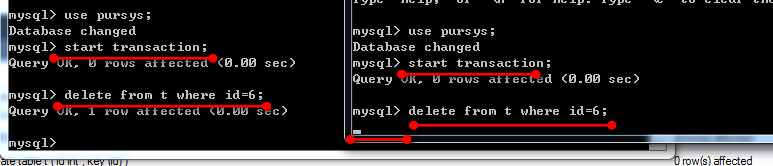### 3.4.2 删除一个不存在的值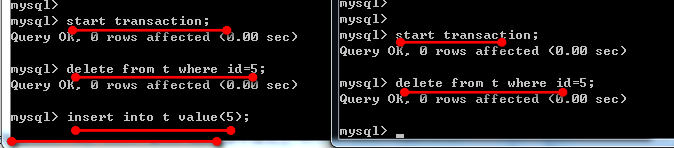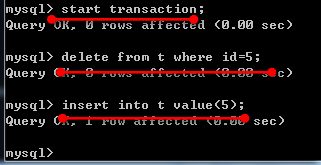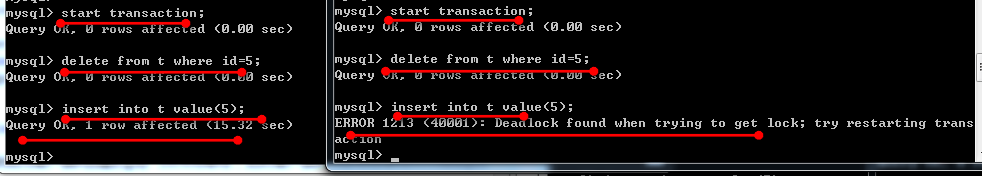# 4 案例演示当前读和快照读

``````create table t (
id int ,
key (id)
);
``````

``````insert into t values(1),(2),(6),(8);
``````

``````start transaction;
select * from t where id>=6;
``````

``````start transaction;
insert into t value(10);
commit;
``````

``````select * from t where id>=6;
select * from t where id>=6 for update;
``````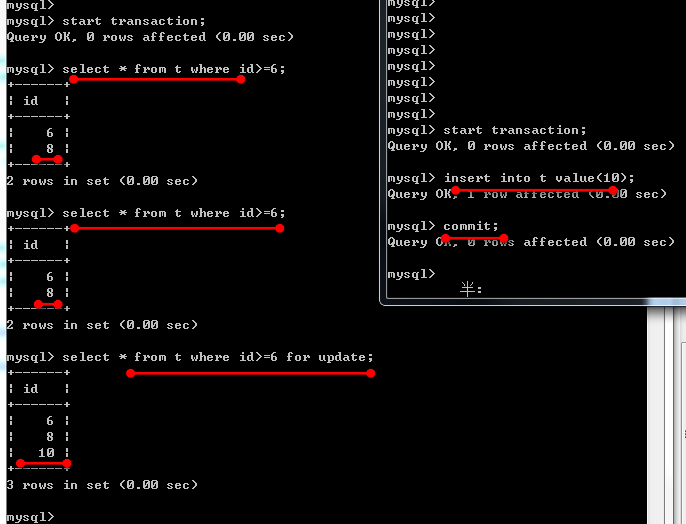+ 关注